# Quantum Computing

Background / Motivation

Quantum computers have the potential to produce tremendous improvements in computational performance for a wide range of problems in various fields, including optimisation, machine learning, chemistry, finance, and healthcare. As the performance of quantum hardware improves, so does the possibility of implementing quantum algorithms that outperform their classical counterparts. There is currently an ongoing race amongst various academic research groups, companies, and national research labs to produce the first demonstration of a practical quantum advantage – a milestone in which a quantum computer solves a problem of practical interest with a performance that classical computers cannot match.

Such a demonstration would involve:
1) using actual quantum hardware to run such a quantum algorithm,
2) showing that classical computers cannot perform as well.

At IHPC, we take a holistic hardware-agnostic full-stack approach that aims to harnesses the power of quantum computers to produce a practical quantum advantage. We aim to develop end-to-end full-stack solutions that bridge the gap between end-users problem statements and quantum-accelerated solutions optimally deployed on near-term quantum hardware by leveraging our existing expertise in HPC modelling and simulations and AI, as well as our vibrant developments in the field of quantum computing.

Together with the Centre for Quantum Technologies (CQT) at the National University of Singapore and the National Supercomputing Centre (NSCC) Singapore, IHPC is also a founding member of the National Quantum Computing Hub (NQCH), one of the three national platforms launched by Singapore's Quantum Engineering Programme (QEP), focusing on developing quantum computing capabilities and exploring applications through industry collaborations. IHPC will contribute our expertise in computational science, high performance computing, artificial intelligence and optimisation to this ecosystem effort. This will include work on algorithms and middleware for academia and industry applications.

Capabilities

Our focus are:

Simulations

• Quantum simulations of many-body quantum physics in chemistry, materials, and quantum optics
• Linear systems and differential equations in fluid dynamics, electromagnetism, and solid mechanics

Optimisation

• Gate and annealing-based optimisation
• Quantum-inspired annealing-based optimisation
• Operation research and structure optimisation
• Optimisation for AI/ML

• Pulse-level programming
• Optimal control protocol for noise reduction
• Hardware characterisation via AI/ML

The horizontal efforts to understand the power and limitations of near-term quantum computers and to lower the entry barrier of quantum computing are:

• Quantum algorithms
• Quantum complexity
• Quantum error mitigation
• Benchmarking against classical algorithms and HPC, and cross-quantum-devices
• Full-stack software engineering and workflow developments

Applications

## Simulations & Optimisation

• Entanglement Ansatz for Solving Ground State Problems in Quantum Many-Body Systems via Variational Quantum Eigensolver

Finding a quantum many-body system's ground state energy and wavefunction is a key problem in quantum physics and chemistry. We investigated the long-range XY model by using the variational quantum eigensolver (VQE) algorithm, a class of quantum/classical hybrid algorithm designed for near term quantum computing devices with classical feedback loops embedded. A typical VQE algorithm can be simply described by the figure shown in Fig 1. We are attempting to design VQE ansatzes (Fig 2) with full and linear entanglement structures consisting of different fundamental building blocks of a quantum algorithm: quantum gates. The design concepts and lesson learned provid us with unique proposition in achieving our aspiration towards practical quantum advantage. (J-B You, D E Koh, J F Kong, W-J Ding, C E Png, L Wu, "Exploring variational quantum eigensolver ansatzes for the long-range XY model", arXiv:2109.00288)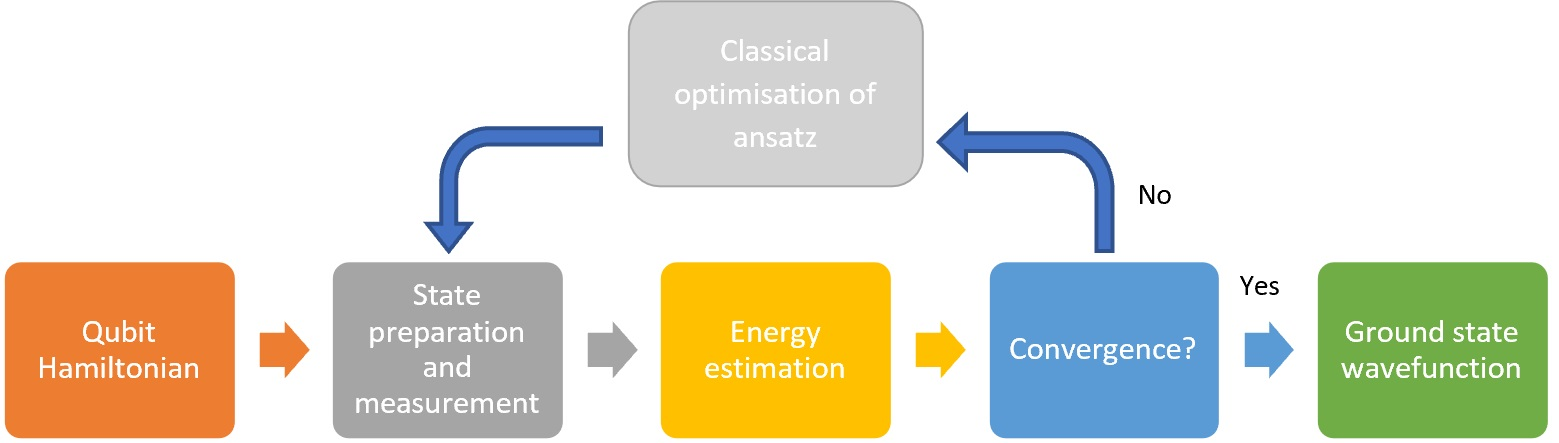Fig 1. Schematics of a typical variational quantum algorithm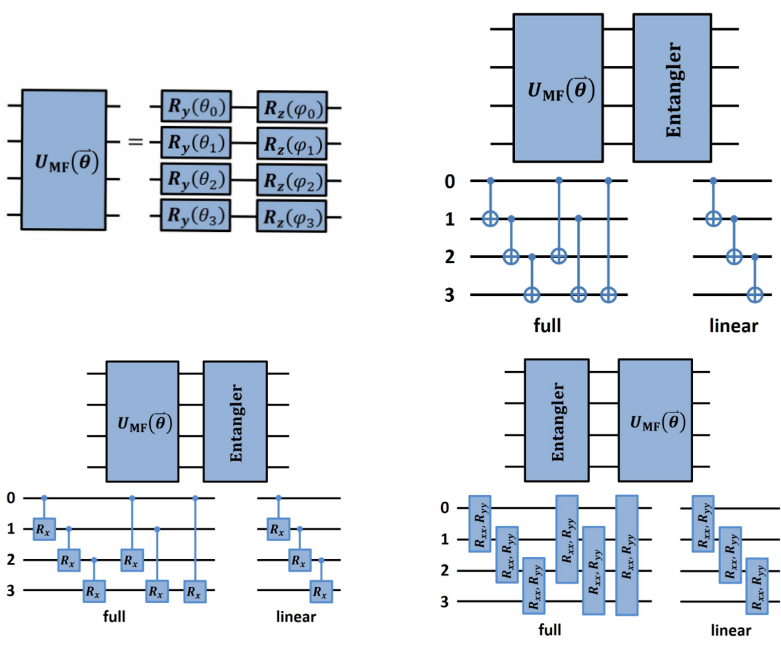Fig 2. Schematics of VQE ansatz design

• Variational Quantum Algorithm (VQA) for Waveguide Propagation Modes

Variational quantum algorithms are one of the most promising methods that can be implemented on noisy intermediate-scale quantum (NISQ) machines to achieve a quantum advantage over classical computers. We have extended the use of a variational quantum algorithm in conjunction with the finite difference method to calculate the propagation modes of an electromagnetic wave in a hollow metallic waveguide (Fig 3). The waveguide propagation mode problem, described by the Helmholtz equation, is approximated by a system of linear equations, whose solutions are expressed in terms of simple quantum expectation values that can be evaluated efficiently on quantum hardware. (W-B Ewe, D E Koh, S T Goh, H-S Chu, C E Png, “Variational Quantum-Based Simulation of Waveguide Modes”, arXiv:2109.12279)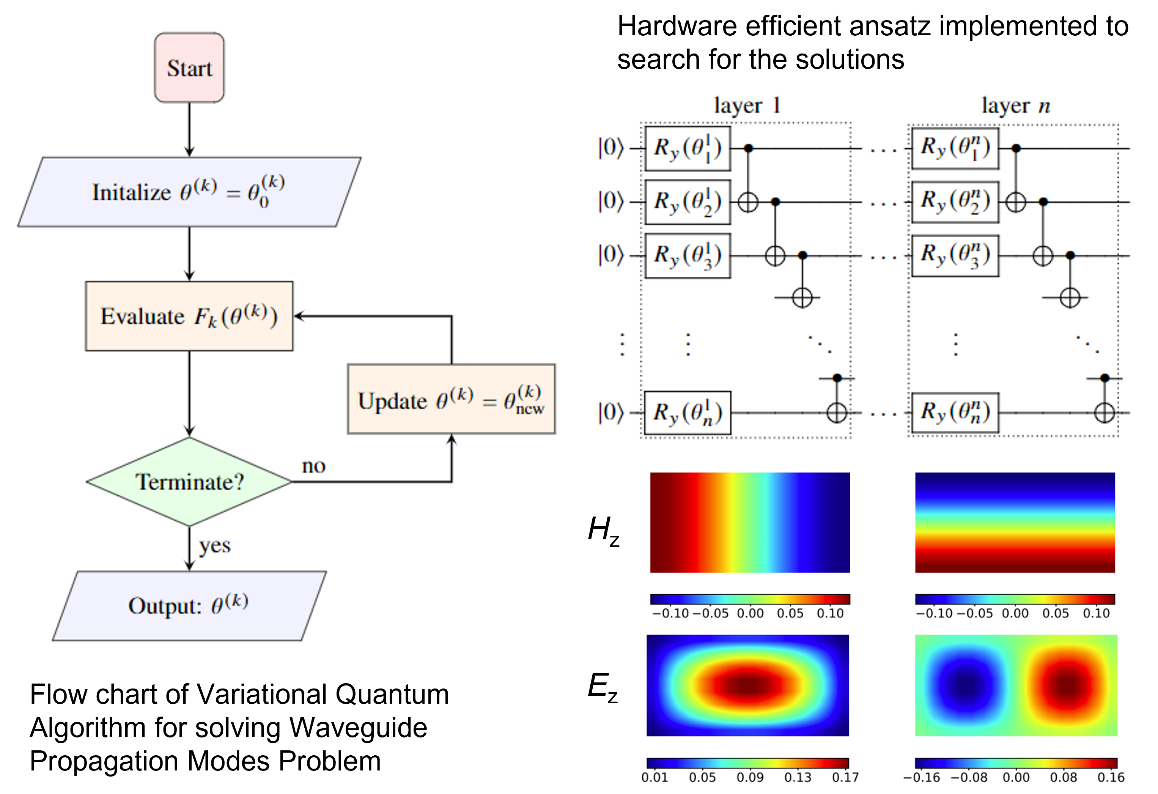Fig 3. Schematics of the variational quantum algorithm workflow, ansatz design and simulated results for a metallic 2D waveguide

• Quantum and Quantum-inspired Annealing-based Optimisation

IHPC's focuses on quantum annealing (QA) research, a promising emerging computing paradigm, and its application to practical use cases. The research topics include but are not limited to Problem formulation, Problem Partitioning, Hyper-parameter tuning and Benchmarking. Closely working with School of Information Systems of SMU and Centre for Quantum Technologies of NUS, IHPC's research contributes towards improving the performance of QA algorithms and methods leveraging NISQ quantum annealing devices and facilitating its application to practical use cases.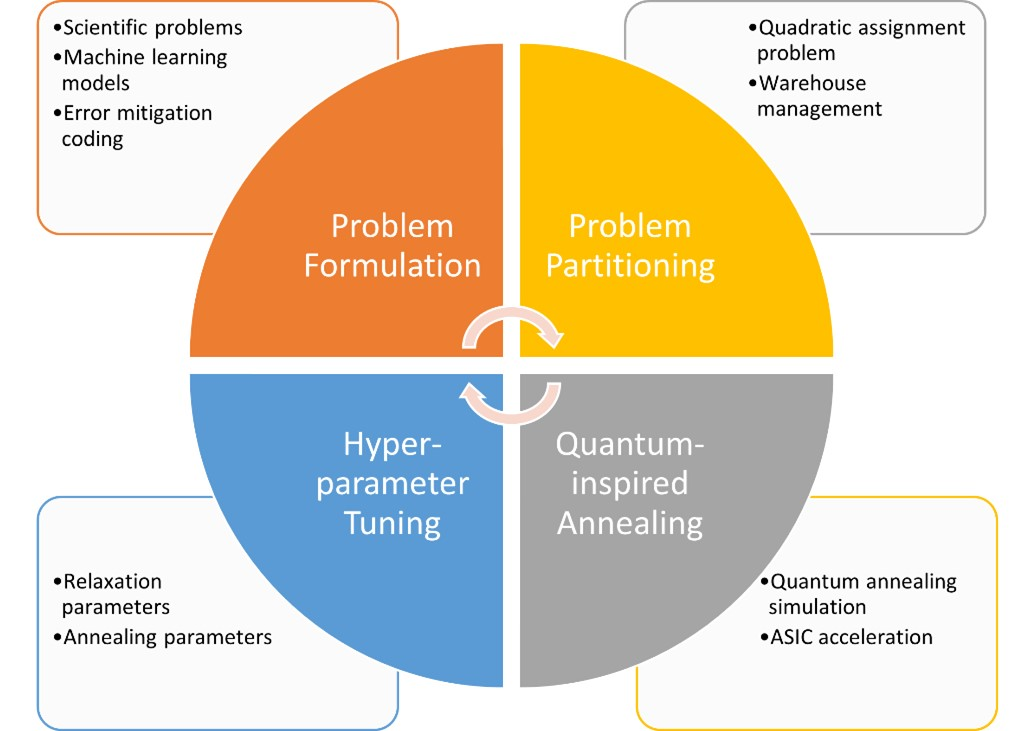• Solving Partial Differential Equations using Variational Quantum Algorithm

Partial differential equations (PDEs) are fundamental to solving important problems ranging from transport phenomena, electromagnetics to finance and social science. The classical-quantum hybrid variational quantum algorithm (VQA) has emerged as a promising strategy for near term noisy quantum devices. Using a parameterised hardware-efficient Ansatz, quantum circuits can be constructed (Fig. 4) to solve, for example, a heat equation through implicit time-stepping with high fidelity. Our algorithm extends economically to higher-order time-stepping schemes and can be adapted to solve other evolution equations, such as reaction-diffusion and incompressible Navier-Stokes equations (Fig. 5).

F.Y. Leong, W.-B. Ewe and D.E. Koh, 2022, “Variational Quantum Evolution Equation Solver”, Sci. Rep. 12, 10817.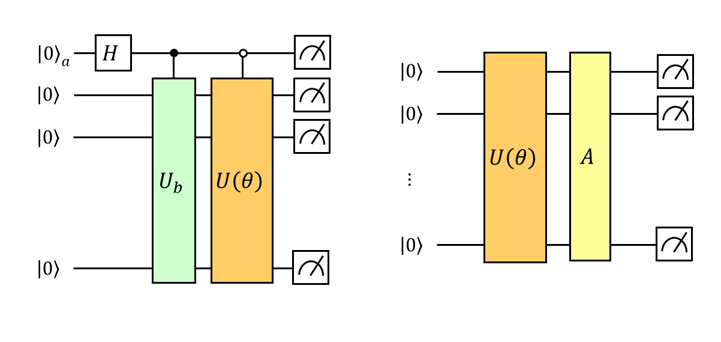Fig 4: Quantum circuits for VQA, where U(θ) is a parameterised Ansatz, Ub and A are unitaries representing the source term and the Hamiltonian respectively.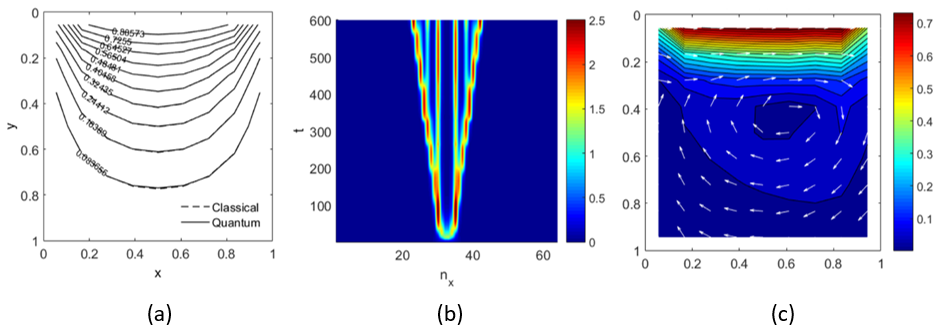Fig 5: High-fidelity VQA solutions to PDEs, such as (a) 2D steady heat conduction (contour plot), (b) Two-component Gray-Scott reaction-diffusion model (space-time plot) and (c) 2D lid-driven cavity flow (quiver plot, Re = 100).

## Quantum algorithms, quantum complexity & quantum error mitigation

• Estimating Quantum Expectation Values using Classical Shadows on Noisy Quantum Computers

The classical shadows protocol (Fig 6) is a hybrid quantum-classical protocol that is used to predict target functions of an unknown quantum state. Unlike full quantum state tomography, the protocol requires only a few quantum measurements to make many predictions with a high success probability and is therefore more amenable to implementation on near-term quantum hardware. In this work, we studied the effects of noise on the classical shadows protocol. We considered the scenario in which the quantum circuits involved in the protocol are subject to various known noise channels and derived an analytical upper bound for the sample complexity in terms of a generalised shadow norm for both local and global noise. By modifying the classical post-processing step of the noiseless protocol, we defined an estimator that remains unbiased in the presence of noise. Our results can be used to prove rigorous sample complexity upper bounds in the cases of depolarising noise and amplitude damping. (D E Koh, S Grewal, "Classical shadows with noise", arXiv:2011.11580)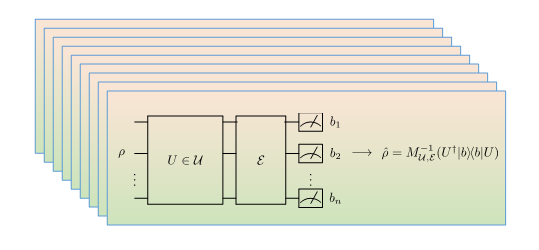Fig 6. Schematic illustrating the (noisy) classical shadows protocol

• Statistical Complexity of Quantum Circuits

We study quantum circuits in terms of their statistical complexity, which is a notion that measures the richness of classes of real-valued functions and provides bounds on the generalisation error associated with learning from training data. These bounds may in turn be used to determine how a hypothesis function may perform on unseen data drawn from an unknown probability distribution. We applied measures of statistical complexity, namely the Rademacher complexity and the Gaussian complexity, to the quantum circuit model in quantum computation and studied how the statistical complexity depends on various quantum circuit parameters (Fig 7). We investigated the dependence of the statistical complexity on the resources, depth, width, and the number of input and output registers of a quantum circuit. To study how the statistical complexity scales with resources in the circuit, we introduced a resource measure of magic based on the (p,q) group norm, which quantifies the amount of magic in the quantum channels associated with the circuit. (K Bu, D E Koh, L Li, Q Luo, Y Zhang, "On the statistical complexity of quantum circuits", arXiv:2101.06154; K Bu, D E Koh, L Li, Q Luo, Y Zhang, "Effects of quantum resources on the statistical complexity of quantum circuits", arXiv:2102.03282; K Bu, D E Koh, L Li, Q Luo, Y Zhang, "Rademacher complexity of noisy quantum circuits", arXiv:2103.03139)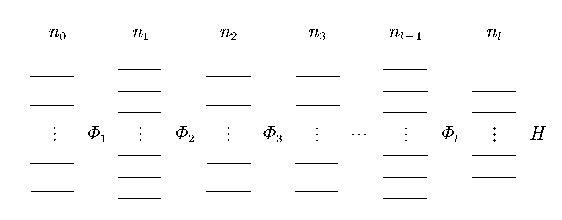Fig 7. Circuit diagram for a class of quantum circuits considered in this work

• Connecting Geometry and Performance of Two-Qubit Parameterised Quantum Circuits

Parameterised quantum circuits (PQCs) are a central component of many variational quantum algorithms, yet there is a lack of understanding of how their parameterisation impacts algorithm performance. We initiate this discussion by using principal bundles to geometrically characterise two-qubit PQCs. On the base manifold, we use the Mannoury-Fubini-Study metric to find a simple equation relating the Ricci scalar (geometry) and concurrence (entanglement).

By calculating the Ricci scalar during a variational quantum eigensolver (VQE) optimisation process, we gained a new perspective to how and why Quantum Natural Gradient outperforms the standard gradient descent. We argue that the key to the Quantum Natural Gradient's superior performance is its ability to find regions of high negative curvature early in the optimisation process. These regions of high negative curvature appear to be important in accelerating the optimisation process. (A Katabarwa, S Sim, D E Koh, P-L Dallaire-Demers, “Connecting geometry and performance of two-qubit parameterized quantum circuits”, arXiv:2106.02593)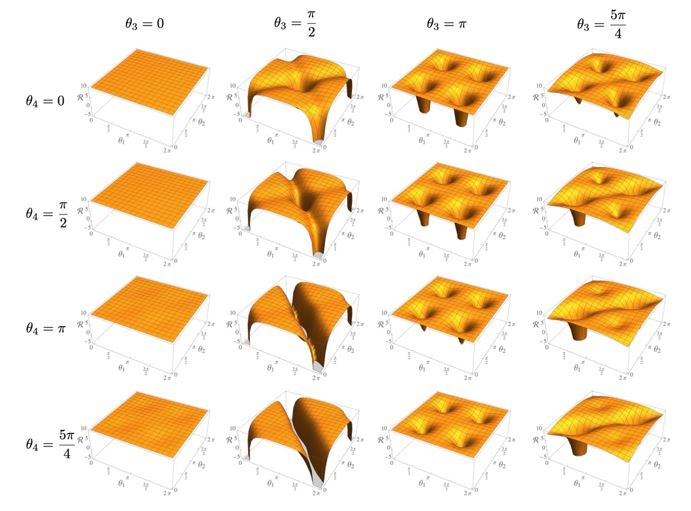Fig 8. Landscapes of scalar curvatures related to various circuit ansatzes

Collaboration Opportunities

IHPC welcomes interested parties to collaborate in research and development relating to Quantum Computing, especially in identifying new technology areas to develop new applications, multi-disciplinary projects, or commercialisation outcomes.